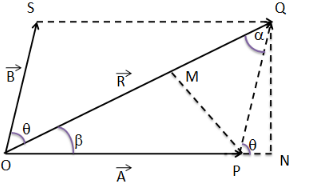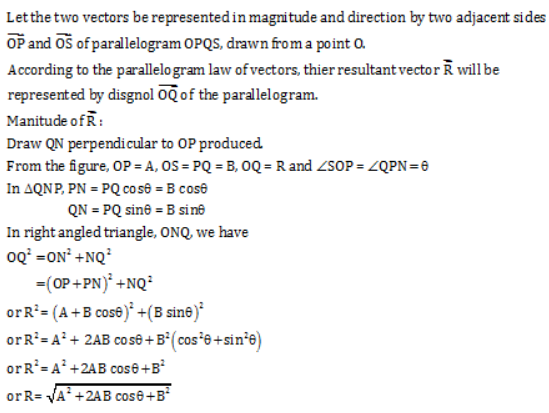# Discuss parallelogram law of vector addition.find the expression for resultant vector using it

discuss parallelogram law of vector addition.find the expression for resultant vector using it.

It states that if two vectors acting on a particle sat the same time be represented in magnitude and direction by the two adjaoent sides of a parallelogram drawn from a point, than their resultant is represented in magnitude and direction by the diagonal of the parallelogram drawn from the same point.